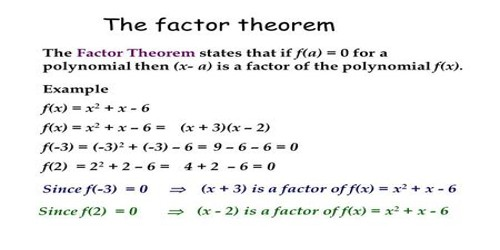Mathematic

# Factor TheoremThe factor theorem is a theorem linking factors and zeros of a polynomial. It is commonly applied to factorizing and finding the roots of polynomial equations. This Theorem is a result of the Remainder Theorem, and is based on the same reasoning. The theorem states that (x – r) is a factor of a polynomial f(x); if f (r) = 0, that is, r is a root of f(x). Factor theorem problems are typically solved by applying synthetic division and then checking for a zero remainder. The remainder theorem is often used with the factor theorem

If the polynomial p(x) is divided by x – α then by division algorithm,

P(x) = (x – α) q(x) + R,

where q(x) is the quotient and R is the remainder which is a constant.

Putting x = α in P(x) = (x – α) q(x) + R, we get,

p(α) = (α – α) q(α) + R

⟹ p(α) = R.

If R = p(α) = 0, then

p(x) = (x – α) q(x) and so (x – α) is a factor of p(x).

x – α is a factor of p(x) if p(α) = 0, and if p(α) = 0 then p(x) has a factor x – α.

The point of the Factor Theorem is the reverse of the Remainder Theorem: If you synthetic-divide a polynomial by x = a and get a zero remainder, then, not only is x = a a zero of the polynomial (courtesy of the Remainder Theorem), but x – a is also a factor of the polynomial (courtesy of the Factor Theorem).

The Factor Theorem states that

(x – a) is a factor of the polynomial f(x) if and only if f(a) = 0

Take note that the following statements are equivalent for any polynomial f(x).

• (x – a) is a factor of f(x).
• The remainder is zero when f(x) is divided by (x – a).
• f(a) = 0.
• The solution to f(x) = 0 is a.
• The zero of the function f(x) is a.

Application of Factor Theorem

Factor Theorem helps us to find factors of polynomial equations, by substituting in number values for x to see whether the equation equals zero. For any polynomial P(x), the factor theorem would state that P(a) = 0, where (x – a) is a factor of P(x).

1. Find the roots of the equation 2x2 – 7x + 6 = 0. Hence factorize 2×22 – 7x + 6.

Solution:

Here, the equation is 2x2 – 7x + 6 = 0

⟹ 2x2 – 4x – 3x + 6 = 0

⟹ 2x(x – 2) – 3(x – 2) = 0

⟹ (x – 2)(2x – 3) = 0

⟹ x – 2 = 0 or 2x – 3 = 0

⟹ x = 2 or x = 3/2

Therefore, 2x2 – 7x + 6 = 2(x – 2)(x – 3/2) = (x – 2)(2x – 3)

1. Find the quadratic equation whose roots are 1 + √3 and 1 – √3.

Solution:

We know that the quadratic equation whose roots are α and β, is

(x – α)(x – β) = 0

Therefore, the required equation is {x – (1 + √3)}{x – (1 – √3)} = 0

⟹ x2 – {1 – √3 + 1 + √3}x + (1 + √3)( 1 – √3) = 0

⟹ x2 – 2x + (1 – 3) = 0

⟹ x2 – 2x – 2 = 0.

Information Source: